# How to make a link between an excel file and AppInventor

I just tried google sheets and it handles the calculations. I am new to google sheets too, How does the connectivity work ?

It’s actually matrix calculations, And I need some functions like iferror to make it work. So doing the calculations on appinventor isn’t possible I think.

For the calculations, if you give an example of what has to be done, our resident maths gurus may offer a solution. It makes more sense to do it on the app, so worth exploring this first.

Some resources / things to look at:

https://puravidaapps.com/math.php

A wider search on matrix:

For data exchange with google sheets, it is a matter of setting the sheet to receive, process and return, and using the web component to handle this. You might need an intermediate script project.

Alright, here’s more details.

I want to make a robotic arm that is controllable with an app via bluetooth.

The data will send coordinates in the X Y Z coordinate system and the google sheet will send back the joint angles of the arm.

I need to do some calculations to know how to move the robot in the x y z coordinate system.

the data in should go in the cells O4, O5, O6, O7, O8, O9 and the data to retrieve would be from the cells Q4, Q5, Q6, Q7, Q8, Q9.

I will take a look at the calculation extensions.

Thank you.

Nothing there in the sheet I can see that can’t be done with the built in maths functions in AppInventor. Obviously easier to see/do on a spreadsheet for a newbiePerhaps the easiest way to send the values is to append a row to another sheet - with the values linked to O4:O9 and to then get the values in Q4:Q9 for the return…something like:

``````doGet(e) {
var sh = ss. getSheetByName("Sheet2");
sh.appendRow([e.parameter.o4,e.parameter.o5,e.parameter.o6,e.parameter.o7,e.parameter.o8,e.parameter.o9]);
var sht = ss. getSheetByName("Sheet1");
var rng = sht.getRange('Q4:Q9');
var vals = rng.getValues();
return ContentService.createTextOutput(vals);
}
``````

(not tested)

In the AppInventor app, you build a url to the sheet, including all the parameters o4 to o9

something like:

`https://script.google.com/macros/s/AKfyJMzcnZgexctQgIcyz_ST8ZdWmQB3l55qM/exec?o4=12345&o5=23456&o6=34567......`

1 Like

Thank you very much for your answer ! There is something I forgot to mention.

The coordinates that i send are only relative to the ones that are in the sheets.

To be clear, the app sends numbers like

+10mm to axis X
-2mm to axis Y
+6° to Yaw

I don’t really know how to do this, but the app needs to :

-> get the current coordinates from the spreadsheet (O4:O9)
-> add or substract the numbers to it
-> send the new coordinates back to the spread sheet (O4:O9)
-> get the joint angles from the spreadsheet.

Clientside, the script should do :
-> send the current coordinates from the spreadsheet to the app (O4:O9)
-> WAIT for the new coordinates to come back from the app
-> get the new coordinates
-> return the new joint angles. (Q4:Q9)

I know the script you wrote does the last two steps, but I don’t know how to get the script to wait for the app to send coordinates first.

If you want to do it entirely in blocks,
you can create a collection of value procedures, one per Excel cell,
each named after the cell (i.e. O23) containing that particular formula.
In the procedure definition, duplicate the calculation from that Excel cell,
using value procedure calls for the cell references.

This will be tedious and dumb, but can be split up among a team
one image per function, and the Block Editor’s secret
Drag and Drop feature.

By the Way, double check the AI2 docs for its trig functions.
Are they in degrees, or in radians?

It is in degrees

https://appinventor.mit.edu/explore/ai2/support/blocks/math#sin

The thing that worries me if I do the spreadsheet in blocks is : how do I make the function iferror() in blocks ?

iferror() can be done using the ifthenelse value block and the
is-number block, and appropriate tests.

There is also a Screen1 error catcher block, but that’s too blunt an instrument.

Here’s a starter app …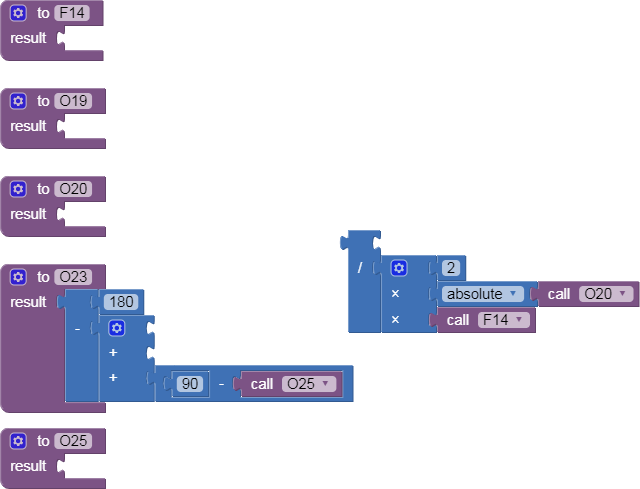kinematics.aia (453.7 KB)

If the blocks are too slow at run time, you might need to switch to
similarly named JavaScript functions in a web page in the Media folder.
Search for ‘FAq Javascript Stunts’ in this forum.
I’m not an expert on that, though.

It seems easier than I thought, It’s just time consuming.

I will make the spreadsheet into blocks and see if it’s too slow.

I’m having trouble making the iferror function.

How do say that the cell O25 is either DEGRES(ATAN(ABS(E16)/F15) or else 90 ?

You used the wrong if/then block.

Use the one with the nipple on the left.

Since the division and the atan() function are the ones that cause an error if inputs are out of bounds,
wrap them with bounds tests on their inputs, like
if F15 = 0 then don’t do the atan but return 90
else do the atan()

Be aware there might be an atan2() block that might not need the division.
Also, I would double check if you need that block that converts radians to degrees.
Does AI2 atan() return degrees already?
Each block has a tool tip visible if you hover over it.

For team coordination, a free github project would make a handy place to share those procedures, one per .png file.

kinematics.aia (454.2 KB)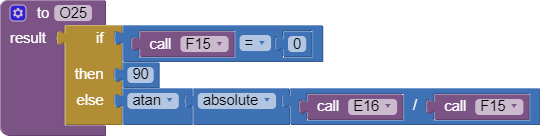After sleeping on this, I suspect you need to add a design layer to
your code with value procedures expressing Excel versions of all the AI2 trig blocks, for use with radians instead of degrees.

(Excel trig tutorial: http://science.clemson.edu/physics/labs/tutorials/excel/trig.html)

This would allow direct transliteration of the Excel formulas into AI2
formulas calling the new layer of value procedures.

Here’s a package for you …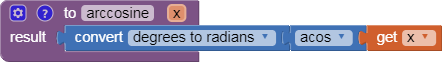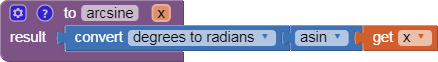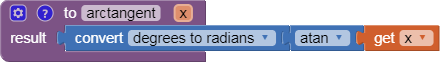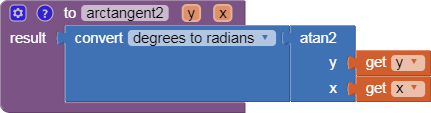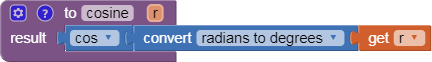Excel_trigonometry.aia (2.3 KB)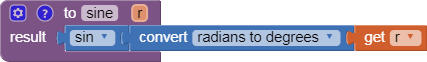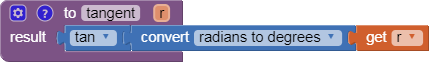This is a trigonometry library for people trying to convert an Excel trigonometry formula to an AI2 trigonometry formula. Everywhere the Excel formula calls a trig function, replace that call in AI2 with a call to the equivalent function in this library. Abe Getzler

Thank you for your input, I will first check if the blocks work with the regular cos and sin functions and use this library in case it doesn’t work.

I just finished making half the spreadsheet in appinventor with AGB’s method and it is very, very slow.

here’s the app if you want to test it : Arduino_Robot_Arm_Control_Forward_Kinematics.aia (261.3 KB)

It takes about 25 seconds for my phone to calculate new coordinates, and this is just the forward kinematics.

Everything works fine until the app calculates the multiplications.

I will just go back to making an app script to use my google spreadsheet.

If you have any ressources I can work with, I will gladly study them !

Sorry AI2’s Math blocks provide a slow calculation. Math block calculation is very SLOW compared to what is possible using javascript or using a compiler like the professional Android Studio.

AI can use javascript to perform calculations. You might use it to do your calculations … here are links to some possible javascript code. Depending on exactly what you need to calculate javascript Finding Angles might help if using a spreadsheet does not pan out. .

see an App Inventor/JavaScript example here https://puravidaapps.com/mathhtml.php

Taifun

Trying to push the limits! Snippets, Tutorials and Extensions from Pura Vida Apps byTaifun.

Hello TimAI2,

Can you give me more detail on how to use your script to read and write to a google sheet ?

I can’t find anywhere how to call a google apps script web app bound to the spreadsheet from app inventor.

Here is your Google Apps Script Webb App code plus some other code I found here :

``````function doGet(e) {

}

// Read from A1 to A6
var sh = ss.getSheetByName("Sheet1");
var rg = sh.getDataRange().getValues();
var outString = '';
for(var row=0; row<7 ; ++row){
outString += rg[row].join(',') + '/n';
}
return ContenrService.createTextOutput(outString);
}

// Write from A1 to A6 and read from B1 to B6
else if(e.parameter.func == "WRITE"){
var sh = ss. getSheetByName("Sheet2");
sh.appendRow([e.parameter.a1,e.parameter.a2,e.parameter.a3,e.parameter.a4,e.parameter.a5,e.parameter.a6]);
var sht = ss. getSheetByName("Sheet1");
var rng = sht.getRange('B1:B6');
var vals = rng.getValues();
return ContentService.createTextOutput(vals);
}
}
``````

Is this code correct ? I don’t really know how to test it.

In app inventor, I would like to :
-> call the READ function of the script
-> call the WRITE function of the script

You also said that I need to build a url to the sheet. I have to use some join text blocks but I cant figure out what part of the link to the script I should write.

My questions are :

What is the url of the script ? (I wrote “something something” istead in the blocks
Am I going the right way ?

Here is my advancement in app inventor :

To finish, I am sorry if there are ressources online which are already answering my questions. I can’t figure out which words to type in google.

Thanks !

Yes, that is my scripting as wellProbably best if I build up a simple example. Need a little while to do that…

Did you build the .apk, or just run it in the Companion?